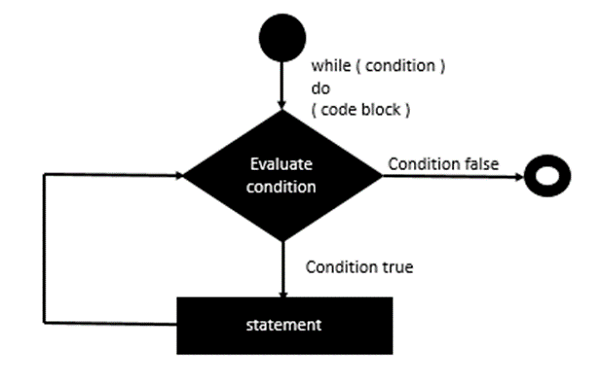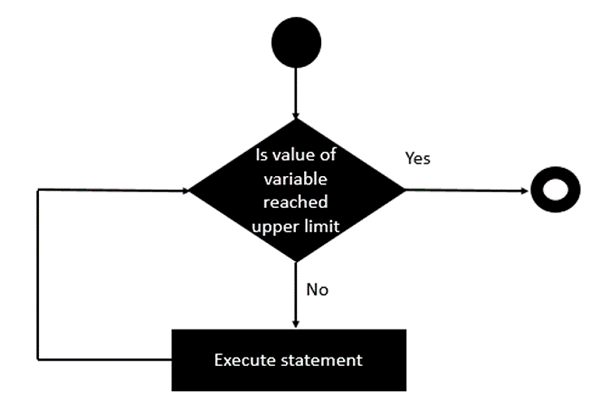# Erlang - Loops

Erlang is a functional programming language and what needs to be remembered about all functional programming languages is that they don’t offer any constructs for loops. Instead, functional programming depends on a concept called recursion.

## while Statement Implementation

Since there is no direct while statement available in Erlang, one has to use the recursion techniques available in Erlang to carry out a while statement implementation.

We will try to follow the same implementation of the while loop as is followed in other programming languages. Following is the general flow which will be followed.Let’s look at an example of how we can use recursion to implement the while loop in Erlang.

### Example

```-module(helloworld).
-export([while/1,while/2, start/0]).

while(L) -> while(L,0).
while([], Acc) -> Acc;

while([_|T], Acc) ->
io:fwrite("~w~n",[Acc]),
while(T,Acc+1).

start() ->
X = [1,2,3,4],
while(X).
```

The following key points need to be noted about the above program −

• Define a recursive function called while which would simulate the implementation of our while loop.

• Input a list of values defined in the variable X to our while function as an example.

• The while function takes each list value and stores the intermediate value in the variable ‘Acc’.

• The while loop is then called recursively for each value in the list.

The output of the above code will be −

```0
1
2
3
```

## for Statement

Since there is no direct for statement available in Erlang, one has to use the recursion techniques available in Erlang to carry out a for statement implementation.

We will try to follow the same implementation of the for loop as is followed in other programming languages. Following is the general flow which should be adhered to.Let’s look at an example of how we can use recursion to implement the for loop in Erlang.

### Example

```-module(helloworld).
-export([for/2,start/0]).

for(0,_) ->
[];

for(N,Term) when N > 0 ->
io:fwrite("Hello~n"),
[Term|for(N-1,Term)].

start() ->
for(5,1).
```

The following key points need to be noted about the above program −

• We are defining a recursive function which would simulate the implementation of our for loop.

• We are using a guard within the ‘for’ function to ensure that the value of N or the limit is a positive value.

• We recursively call the for function, by reducing the value of N at each recursion.

The output of the above code will be −

```Hello
Hello
Hello
Hello
Hello
```